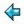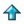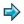### refoliate.m

```> tree ::= Leaf num | Fork tree tree        ||literate script
```
PROBLEM: write down the definition of a function which takes a tree and returns a tree of the SAME SHAPE, containing the same data, but with the leaves moved around so that the data appears in ascending order, when the tree is scanned from left to right.
```> reorder :: tree->tree
> reorder t = refoliate t (sort (fringe t))
```
Our idea here is that `fringe' extracts a list of all the data in the tree, while `refoliate' takes a tree and a list of data, and replaces the leaves of the tree with the given data, preserving the shape of the tree. We define fringe first, as it is the easiest.
```> fringe :: tree->[num]
> fringe (Leaf n) = [n]
> fringe (Fork s t) = fringe s ++ fringe t
```
Aside - there is a trivial change to the last line which alters the behaviour of fringe so that the call to sort is no longer necessary. We can replace `++' by a call to the library function `merge'. This would improve the efficiency of the solution.

We define `refoliate' in terms of an auxiliary function which takes a subtree and the list of replacement data, and returns a pair - the refoliated subtree, and the unused part of the list.

```> refoliate :: tree->[num]->tree
> refoliate t x = fst (refol t x)

> refol :: tree->[num]->(tree,[num])
> refol (Leaf n) (a:x) = (Leaf a,x)
> refol (Fork s t) x = (Fork s1 t1,x'')
>                      where
>                      (s1,x') = refol s x
>                      (t1,x'') = refol t x'
```
Here is an example tree on which to call `reorder', to see that the algorithm works.
```> t1 = mktree [19,0,17,2,15,4,13,6,11,8,9,10,7,12,5,14,3,16,1,18]
```
mktree takes a list and builds a (well-balanced) tree from it.
```> mktree :: [num]->tree
> mktree [] = error "cannot have empty tree"
> mktree [a] = Leaf a
> mktree x = Fork (mktree (take n x)) (mktree (drop n x))
>            where n = # x div 2
```
Finally, we define a function same_shape, which can be used to confirm that reorder holds the shape constant.
```> same_shape :: tree->tree->bool
> same_shape (Leaf a) (Leaf b) = True
> same_shape (Fork s t) (Fork s1 t1) = same_shape s s1 & same_shape t t1
> same_shape s t = False ||all other cases

> test = same_shape t1 (reorder t1)
```